Get instant live expert help with Excel or Google Sheets“My Excelchat expert helped me in less than 20 minutes, saving me what would have been 5 hours of work!”

#### Post your problem and you’ll get Expert help in seconds.

Your message must be at least 40 characters
Our professional Expert are available now. Your privacy is guaranteed.

# Excel ISNONTEXT functionRead time: 20 minutes

While working with Excel, it is fairly easy to determine if a value is not text by using the ISNONTEXT function.  ISNONTEXT belongs to the IS functions, which returns logical values TRUE or FALSE.  This step by step tutorial will assist all levels of Excel users in the usage and syntax of ISNONTEXT function.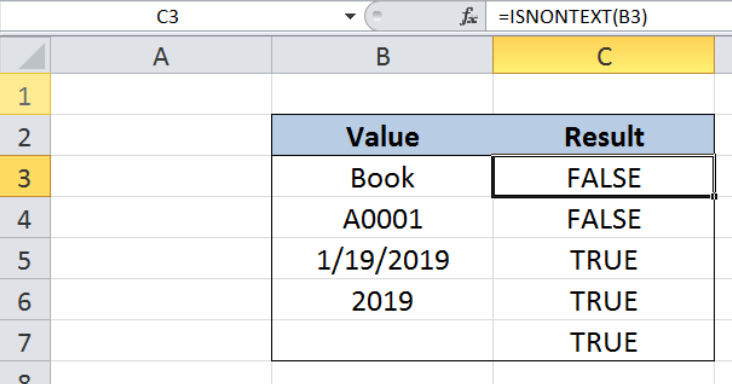Figure 1. Final result: Excel ISNONTEXT function

## Syntax of the ISNONTEXT Function

ISNONTEXT function tests if a value is not text and returns TRUE; otherwise it returns FALSE

`=ISNONTEXT(value)`

• value – the value that we want to test;  value argument can be a blank or empty cell, error or logical value, text, date, number or a cell reference
• ISNONTEXT returns TRUE for non-text values such as date, time, number and empty or blank cells
• Otherwise, it returns FALSE for all text values

## Setting up our Data

Our data consists of two columns:  Value (column B) and Result (column C).  We want to test the value in column B if it is not text, and record the result in column C.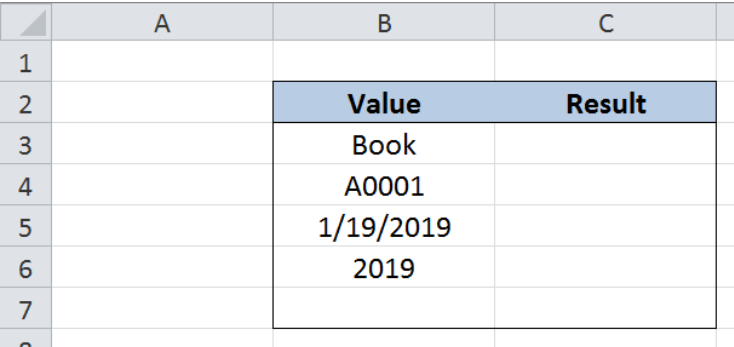Figure 2. Sample data to determine if values are not text

## Test if value is not text

In order to test if a certain value is not text by using the ISNONTEXT function, let us follow these steps:

Step 1.  Select cell C3

Step 2.  Enter the formula: `=ISNONTEXT(B3)`

Step 3.  Press ENTER

Step 4:  Copy the formula in cell C3 to cells C4:C7 by clicking the “+” icon at the bottom-right corner of cell C3 and dragging it down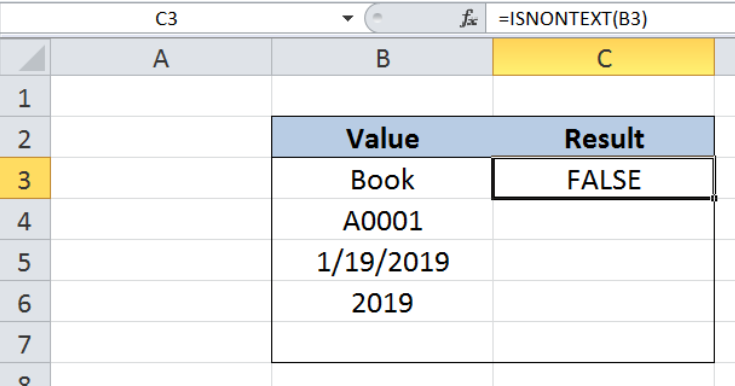Figure 3. Entering the ISNONTEXT formula

The value in B3 “Book is a text string.  When tested using the ISNONTEXT function, it naturally returns FALSE because it is a text value.  The ISNONTEXT returns FALSE for all text values.

In the same manner, cell B4 containing the value “A0001” is also evaluated as FALSE by the ISNONTEXT function because it is a text value.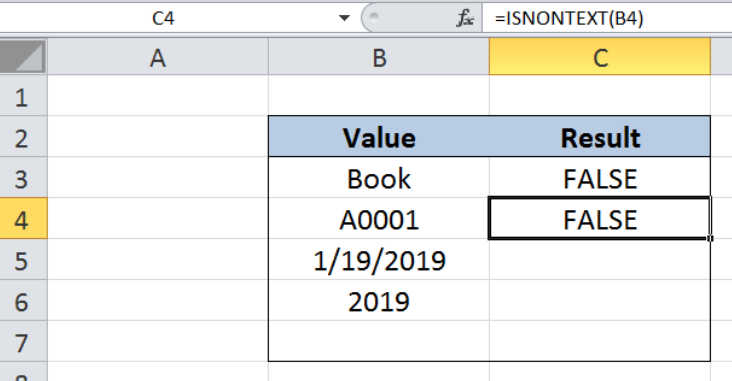Figure 4. ISNONTEXT evaluates text values as FALSE

Cells B5, B6 and B7 contain a date, a number and an empty string, respectively.  These three values are non-text values. Hence, the ISNONTEXT function returns TRUE for these three cells, as shown below.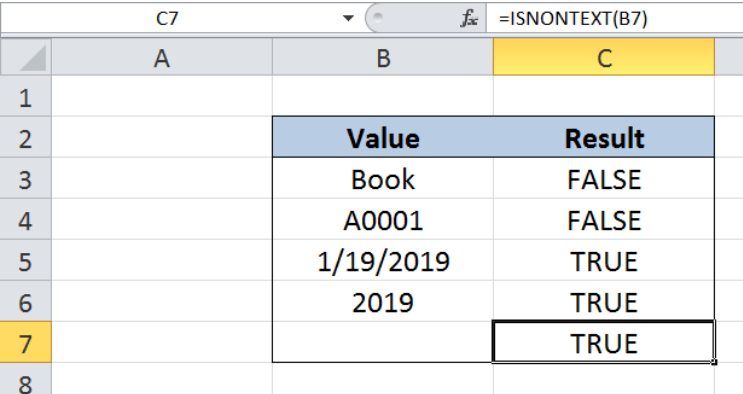Figure 5. ISNONTEXT evaluates non-text values as TRUE

## Enter non-text values as text

There is, however, a special case when dates, times or numbers are evaluated as FALSE by the ISNONTEXT function.  This happens when we enter these values as text values. There are two ways to enter any value as text:

• Input the value with an apostrophe at the start of the text string; see example below where the date is entered as ‘1/19/2019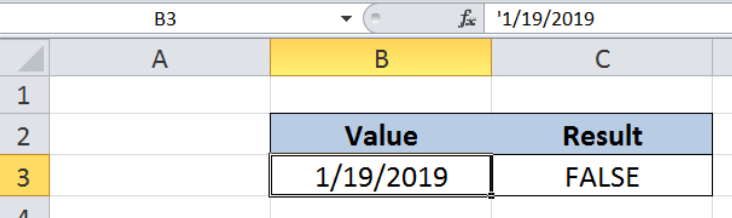Figure 6. Entering non-text value as text using an apostrophe

• Change the format of the cell to Text before inputting the value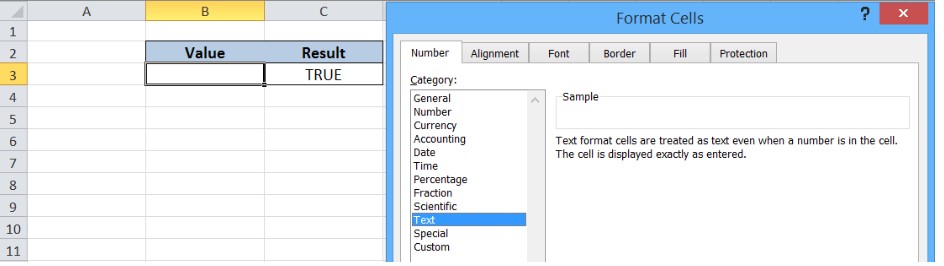Figure 7. Changing cell format to text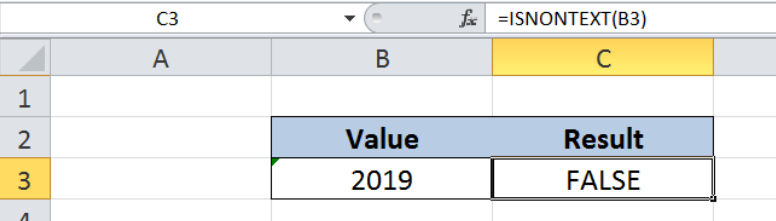Figure 8 . ISNONTEXT returns FALSE for numerical value formatted as text

Most of the time, the problem you will need to solve will be more complex than a simple application of a formula or function. If you want to save hours of research and frustration, try our live Excelchat service! Our Excel Experts are available 24/7 to answer any Excel question you may have. We guarantee a connection within 30 seconds and a customized solution within 20 minutes.

Solution examplesUse the Vlookup Function to complete the "employee" column of table 2. Use "job Id" from table 2 as your lookup_value(s) and table 1 as your reference.
Solved by C. H. in 16 minsIf a cell in another sheet is populated I need a vlookup done. If the cell is not populated I need the cell to return blank.
Solved by T. D. in 60 minsI am trying to make a chart that turns a week range red if nothing is entered in the range. If something is entered then I would like it to turn green. Please Help
Solved by E. U. in 43 minsI need a check box to show/hide an answer of an if function
Solved by Z. U. in 23 minsI need a formula to compare the data in two columns and then export the mismatched data in the 3rd column
Solved by S. Q. in 20 mins